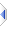# compare

 Definition: integer i = compare(object x1, object x2) Description: Return 0 if objects x1 and x2 are identical, 1 if x1 is greater than x2, -1 if x1 is less than x2. Atoms are considered to be less than sequences. Sequences are compared "alphabetically" starting with the first element until a difference is found. Note that RDS Eu/OpenEuphoria typically need equal() or compare() on each and every non-trivial conditional test, whereas phix does not and can use =, <=, etc. Apart from the obvious legacy code and compatibility with RDS Eu/OpenEuphoria, compare is also very useful in sort routines and the like. Example 1: ```x = compare({1,2,{3,{4}},5}, {2-1,1+1,{3,{4}},6-1}) -- identical, x is 0 ``` Example 2: ```if compare("ABC", "ABCD") < 0 then -- -1 -- will be true: ABC is "less" because it is shorter end if ``` Example 3: ```x = compare({12345, 99999, -1, 700, 2}, {12345, 99999, -1, 699, 3, 0}) -- x will be 1 because 700 > 699 ``` Example 4: ```x = compare('a', "a") -- x will be -1 because 'a' is an atom -- while "a" is a sequence ``` Implementation: Implemented as %opScmp in builtins\VM\pJcc.e (an autoinclude) - be warned however it is low-level complicated stuff that you do not need to know. See Also: equal, min, max, relational operators, sequence operations